Calculation based on multiple choice

HI I am trying to use a formula in a cell that is based on the selcetion of a multipe choice in another cell..   ie multiple choice could be a)5% or b)10%.....  from this,  in another cell I want to multiply a value based on the selection from the multiple choice...

I can get it to work as long as I use a txt box but cant get the formula to recognise the multiple choice value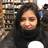Hi Ivor,

--------

'Input Number' * number(chosen('Discount (%)')) / 100

--------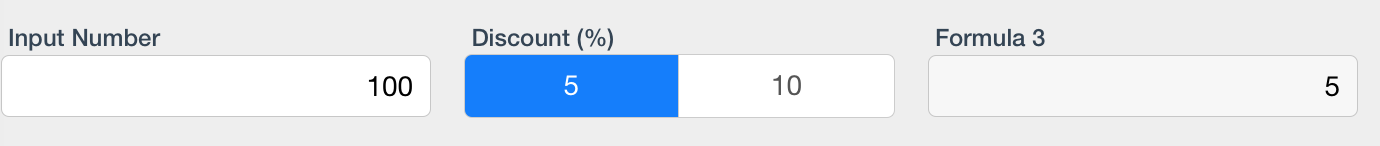Regards,

Sakshi-Ninox Support

Hi thanks for your feedback,  could you expalin a little more I stilll dont understand this formula

where/what is the NUMBER before thr brackets

assume "input number" is the cell that I am trying to apply the discount to ?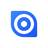That "5" and "10" are the labels of the multiple choice fields are are handled an string. number() is used to convert them to - numbers.

Birger

thanks I now have the formula making the calculation at least but the calcualtion result is wrong (formula below)

It is using the value from the multipe choice as opposed to the actual selection

for example 5% = 1 ,  10%=2  how do I make 5% = 5 and 10% = 10

cost * number('Discount %')Hi Ivor,

The formula is:

-------------

Cost * number(chosen('Discount %')) / 100

-------------

Please input only numbers in Multiple choice field, without the percenage symbol.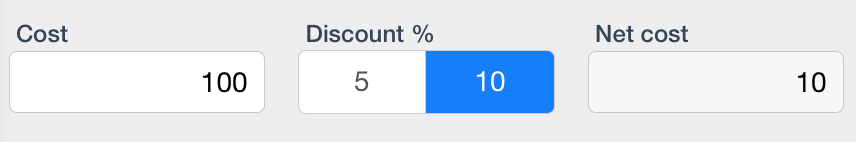Regards,

Sakshi-Ninox Support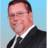Make sure you are using a CHOICE field.. not a MULTIPLE CHOICE field..  number(chosen('MULTIPLE CHOICE")) always returns zero.